# Math in Focus Grade 4 Chapter 7 Practice 1 Answer Key Understanding Tenths

Practice the problems of Math in Focus Grade 4 Workbook Answer Key Chapter 7 Practice 1 Understanding Tenths to score better marks in the exam.

## Math in Focus Grade 4 Chapter 7 Practice 1 Answer Key Understanding Tenths

Shade the squares to represent each decimal.
Each square represents 1 whole.

Example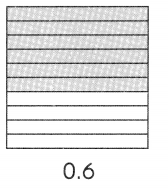Question 1.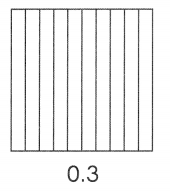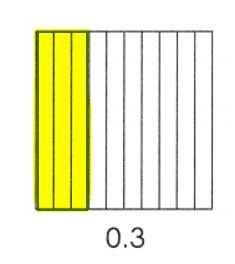Explanation:
0.3 = 3 ÷ 10.

Question 2.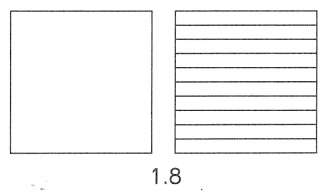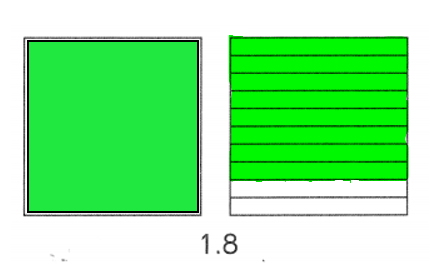Explanation:
1.8 = 18 ÷ 10.

Question 3.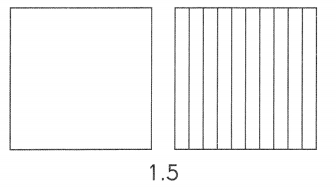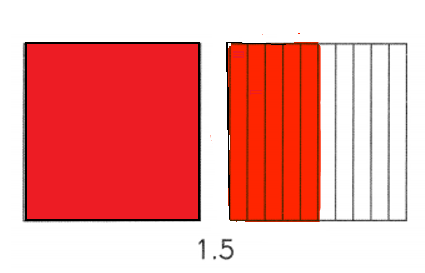Explanation:
1.5 = 15 ÷ 10.

Write the correct decimal in each box.
Question 4.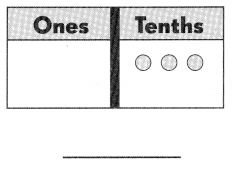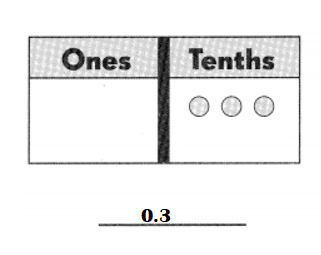Explanation:
0 ones 3 tenths = 0.3.

Question 5.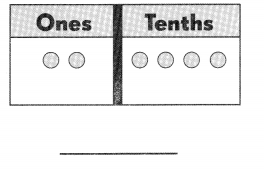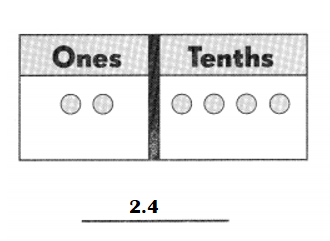Explanation:
2 ones 4 tenths = 2.4.

Question 6.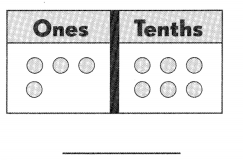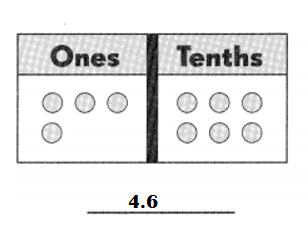Explanation:
4 ones 6 tenths = 4.6.

Question 7.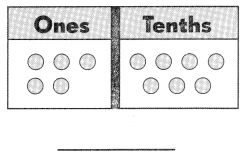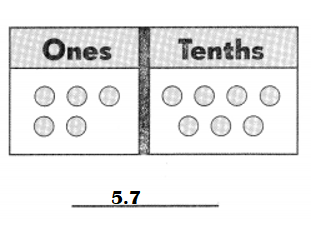Explanation:
5 ones 7 tenths = 5.7.

Write the correct decimal in each box.
Question 8.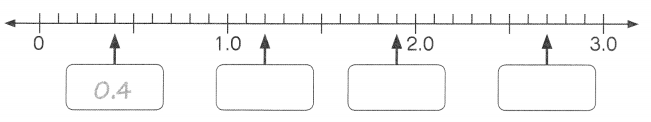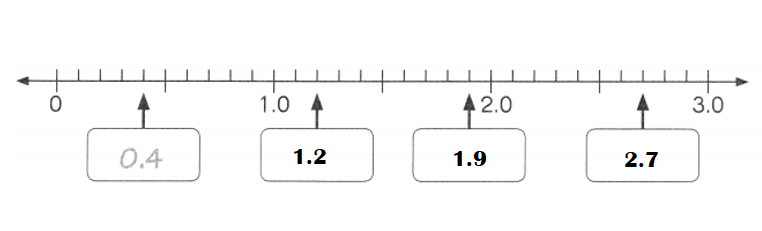Explanation:
1.0 + 0.2 = 1.2.
1.2 + 0.7 = 1.9.
2.0 + 0.7 = 2.7.

Mark ✗ to show where each decimal is located on the number line, Label its value.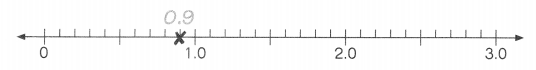Question 9.
1.6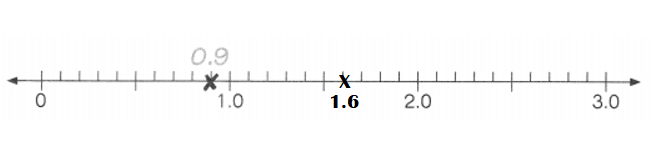Explanation:
1.6 = 1.0 + 0.6.

Question 10.
1.8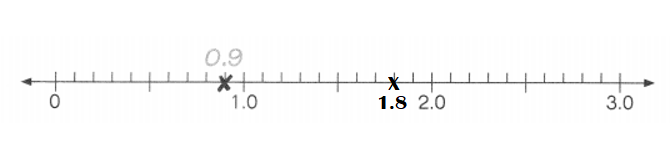Explanation:
1.8 = 1.0 + 0.8.

Question 11.
2.4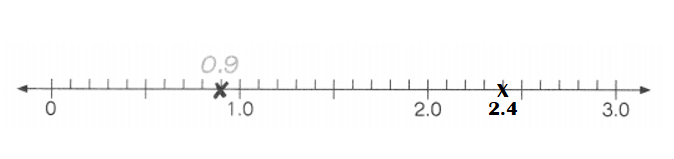Explanation:
2.4 = 2.0 + 0.4.

Write each of these as a decimal.

Question 12.
9 tenths = ___
9 tenths = 0.9.

Explanation:
9 tenths = 9 ÷ 10 = 0.9.

Question 13.
13 tenths = ____
13 tenths = 1.3.

Explanation:
13 tenths = 13 ÷ 10 = 1.3.

Question 14.
26 tenths = ___
26 tenths = 2.6.

Explanation:
26 tenths = 26 ÷ 10 = 2.6.

Question 15.
9 ones and 3 tenths = ___
9 ones and 3 tenths = 9.3.

Explanation:
9 ones and 3 tenths = 93 ÷ 10 = 9.3.

Write each fraction or mixed number as a decimal.
Question 16.
$$\frac{7}{10}$$ = ___
$$\frac{7}{10}$$ = 0.7.

Explanation:
$$\frac{7}{10}$$ = 0.7.

Question 17.
2$$\frac{3}{10}$$ = ___
2$$\frac{3}{10}$$ = 2.3.

Explanation:
2$$\frac{3}{10}$$ = (20 + 3) ÷ 10
= $$\frac{23}{10}$$
= 2.3.

Question 18.
$$\frac{41}{10}$$ = ___
$$\frac{41}{10}$$ = 4.1.

Explanation:
$$\frac{41}{10}$$ =  4.1.

Question 19.
$$\frac{109}{10}$$ = ___
$$\frac{109}{10}$$ = 10.9.

Explanation:
$$\frac{109}{10}$$ = 10.9.

Write each decimal in tenths.
Question 20.
0.3 = ___ tenths
0.3 = 3 tenths.

Explanation:
0.3 = 3 tenths.

Question 21.
5.7 = ___ tenths
5.7 = 57 tenths.

Explanation:
5.7 = 5 ones 7 tenths.

Question 22.
26.1 = __ tenths
26.1 = 261 tenths.

Explanation:
26.1 = 2 tens 6 ones 1 tenths.

Question 23.
48.9 = ___ tenths
48.9 = 489 tenths.

Explanation:
48.9 = 4 tens 8 ones 9 tenths.

Write a fraction and decimal for each measure.
Example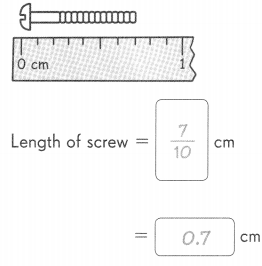Question 24.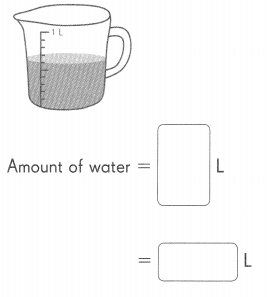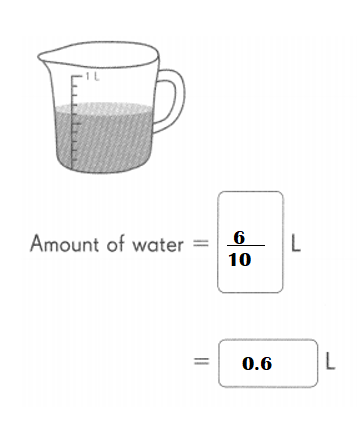Explanation:
Amount of water = 6 ÷ 10L.

Write a mixed number and decimal for each measure.
Question 25.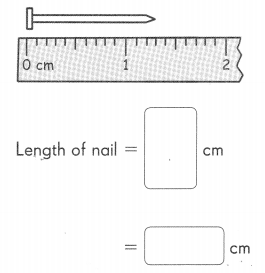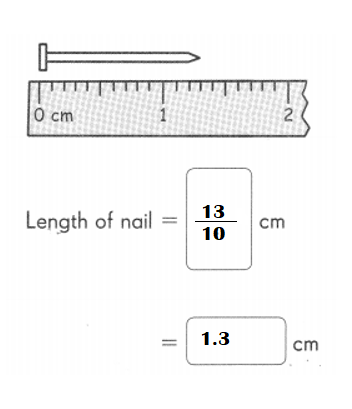Explanation:
Length of the nail = 1.3 cm.

Question 26.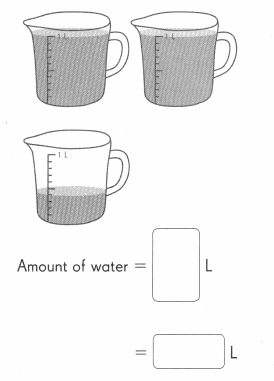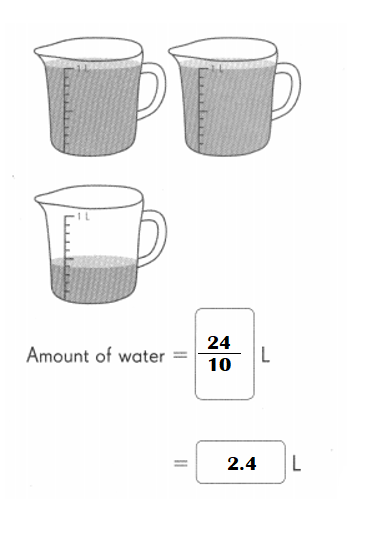Explanation:
Amount of water = 2L + 0.4 L = 2.4 L.

Fill in the blanks:
Question 27.
3.4 = 3 ones and ___ tenths
3.4 = 3 ones and 4 tenths.

Explanation:
3.4 = 3 ones and 4 tenths.

Question 28.
5.8 = ___ ones and 8 tenths
5.8 = 5 ones and 8 tenths.

Explanation:
5.8 = 5 ones and 8 tenths.

Question 29.
22.1 = 2 tens 2 ones and ___ tenth
22.1 = 2 tens 2 ones and 1 tenth.

Explanation:
22.1 = 2 tens 2 ones and 1 tenth.

Question 30.
36.7 = ___ tens 6 ones and 7 tenths
36.7 = 3 tens 6 ones and 7 tenths.

Explanation:
36.7 = 3 tens 6 ones and 7 tenths.

You can write 15.2 in expanded form as 10 + 5 + $$\frac{2}{10}$$. Complete in the same way.
Question 31.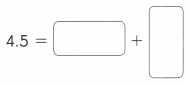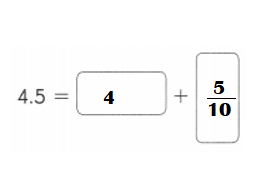Explanation:
4.5 = 4 +  $$\frac{5}{10}$$.

Question 32.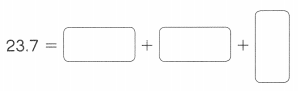Explanation:
23.7 = 20 + 3 +  $$\frac{7}{10}$$.

You can write 14.3 in expanded form as 10 + 4 + 0.3. Complete in the same way.
Question 33.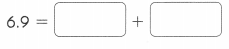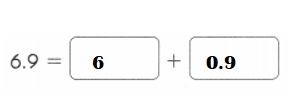Explanation:
Expanded form of 6.9 = 6 + 0.9.

Question 34.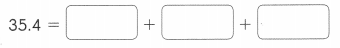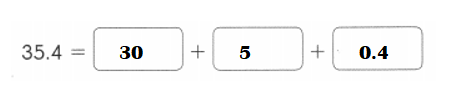Explanation:
Expanded form of 35.4 = 30 + 5 + 0.4.

Fill in the blanks.
Question 35.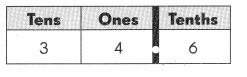The digit 6 is in the ___ place. Its value is ___
3 tens 4 ones 6 tenths = 34.6.

Explanation:
The digit 6 is in the tenths place.
Its value is 0.6.Question 36.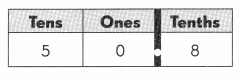The digit 0 is in the ___ place. Its value is ___